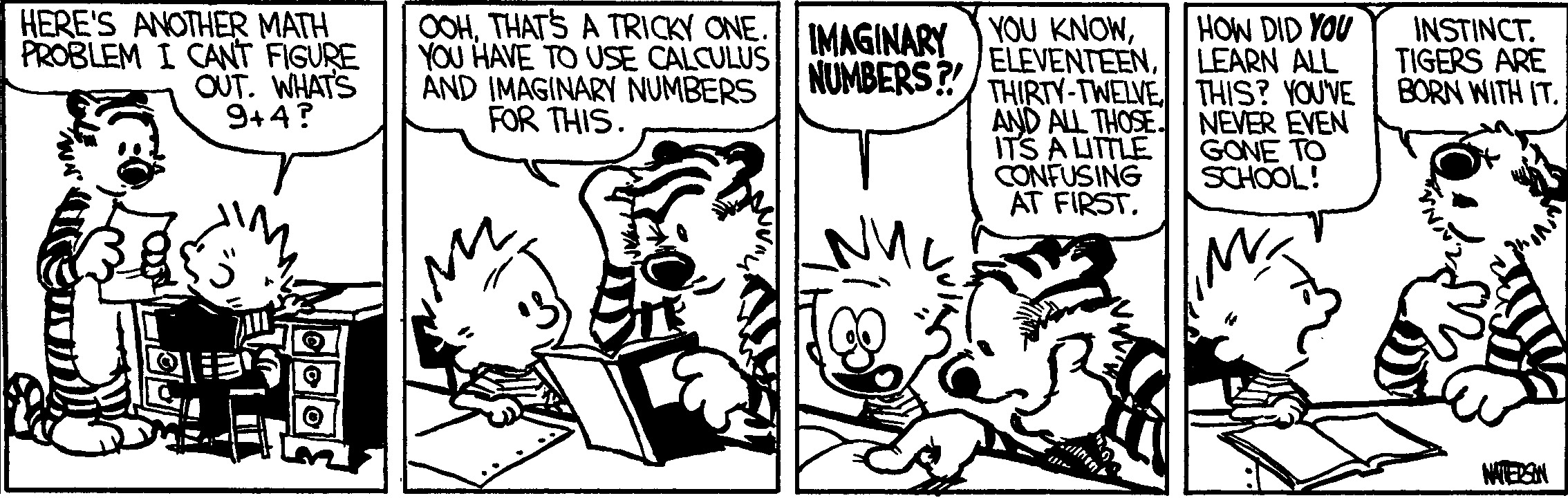This semester, Spring 2022, I am not teaching

Teaching Resources

Matlab - All Tufts students have access to Matlab in the ITS Computing Center @ Eaton Hall. You can also install Matlab on your peronsal computer via the Tufts site license. To obtain the license and install Matlab see the following link. For resources on Matlab, see below.
Python - Python is also a useful tool to learn, along with numpy/scipy/matplotlib. It also happens to be free!

The Mathematical Contest in Modeling (MCM) - This is a 4 day team competition held every year in late January or early Februrary. For more information, see the MCM website, and please see me at some point during the semester if you are interested.
1. Understand the underlying concepts, not just the procedure for getting the answer. Learn to learn!

2. Ask questions if you don't understand. Don't be afraid to visit me during office hours, where we can go over material and homework you don't quite understand.

3. Use the textbook (if there is one). It contains a lot of useful information and pretty pictures. You paid a lot for it most likely, so you might as well read it!

4. Come to class! I don't take attendance, but that's where the learning takes place.

5. Read the ENTIRE syllabus, ALL directions on exams, and any announcements or emails I send out. Most of the time, the information you need has already been given.

6. Mathematics is a language used to describe the physical world. You may already know the specific concepts or will learn new ones that you can apply to all sorts of problems. Keep this in mind and understand that it might just be the question that is posed differently, but the answer is something you already know!

7. Sleep! It's the number one way to improve your mathematics ability. Just not during class...

8. Mathematics jokes are welcome at any time during class. Please keep it appropriate though...
Past Courses
• Tufts University

• MATH 036 - Applied Calculus II

• MATH 042 - Calculus III: Multivariable Calculus

• MATH 051 - Differential Equations

• MATH 087 - Mathematical Modeling and Computation

• MATH 125 - Numerical Analysis

• MATH 220 - Top Ten Algorithms of the 20th Century

• MATH 250 - Seminar in Computational and Applied Mathematics

• MATH 255 - Partial Differential Equations I

• MATH 256 - Nonlinear Partial Differential Equations

• MATH 257 - Numerical Partial Differential Equations

• MATH 293 - Reading Courses in:
• Numerical PDEs, Finite Elements, Multigrid

• Penn State

• MATH 140 - Calculus with Analytic Geometry I

• MATH 141 - Calculus with Analytic Geometry II

• MATH 230 - Calculus and Vector Analysis

• CU Boulder

• APPM 1350 - Calculus I

• APPM 1360 - Calculus II

• GEEN 1360 - Calculus II Workshop

• APPM 2350 - Calculus III

• APPM 2360 - Differential Equations

• APPM 2450 - Calculus III Lab

• APPM 5600 - Introduction to Numerical Analysis I

• APPM 7400 - Teaching and Research Excellence